## Diary — October 2021

#### October 1, 2021I think this print is by Takeji Asano. The glowing lights in the houses and especially the boat are very inviting amid the darkness. You want to go inside.

#### October 3, 2021

Here's a bit of basic stuff about maximal ideals versus prime ideals. Summary: when we first start learning algebra we like fields, so we like maximal ideals. But as we grow wiser, we learn the power of logically simpler concepts.

I'll use 'ring' to mean 'commutative ring'.

The continuous real-valued functions on a topological space form a ring $$C(X)$$, and the functions that vanish at one point form a maximal ideal $$M$$ in this ring. This makes us want 'points' of a ring to be maximal ideals.

Indeed, it's all very nice: $$C(X)/M$$ is isomorphic to the complex numbers $$\mathbb{C}$$, and the quotient map $$C(X) \to C(X)/M \cong \mathbb{C}$$ is just evaluating a function at a point.

Even better, if $$X$$ is a compact space every maximal ideal $$M \subset C(X)$$ consists of functions that vanish at some point in $$X$$. If furthermore $$X$$ is also Hausdorff, the choice of this point is unique.

In short, points of a compact Hausdorff space $$X$$ are just the same as maximal ideals of $$C(X)$$. So we have captured, using just algebra, the concept of 'point' and 'evaluating a function at a point'.

The problem starts when we try to generalize from $$C(X)$$ to other commutative rings.

At first all seems fine: for any ideal $$J$$ in any ring $$R$$, the quotient $$R/J$$ is a field if and only if $$J$$ is maximal. And we like fields — since we learned linear algebra using fields.

But then the trouble starts. Any continuous map of spaces $$X \to Y$$ gives a ring homomorphism $$C(Y) \to C(X)$$. This is the grand duality between topology and commutative algebra! So we'd like to run it backwards: for any ring homomorphism $$R \to S$$ we a map sending 'points' of $$S$$ to 'points' of $$R$$.

But if we define 'points' to be maximal ideals it doesn't work. Given a homomorphism $$f \colon R \to S$$ and a maximal ideal $$M$$ of $$S$$, the inverse image $$f^{-1}(M)$$ is an ideal of $$R$$, but not necessarily a maximal ideal!

Why not?

To tell if an ideal is maximal you have to run around comparing it with all other ideals! This depends not just on the ideal itself, but on its 'environment'. So $$M$$ being maximal doesn't imply that $$f^{-1}(M)$$, living in a completely different ring, is maximal.

In short, the logic we're using to define 'maximal ideal' is too complex! We are quantifying over all ideals in the ring, so the concept we're getting is very sensitive to the whole ring — so maximal ideals don't transform nicely under ring homomorphisms.

It turns out prime ideals are much better. An ideal $$P$$ is prime if it's not the whole ring and $$ab \in P$$ implies $$a \in P$$ or $$b \in P$$.

Now things work fine: given a homomorphism $$f \colon R \to S$$ and a prime ideal $$P$$ of $$S$$, the inverse image $$f^{-1}(P)$$ is a prime ideal of $$R$$.

Why do prime ideals work where maximal ideals failed?

It's because checking to see if an ideal is prime mainly involves checking things within the ideal — not its environment! None of this silly running around comparing it to all other ideals.

And we also get a substitute for our beloved fields: integral domains. An integral domain is a ring where if $$ab = 0$$, then either $$a = 0$$ or $$b = 0$$. For any ideal $$J$$ in any ring $$R$$, the quotient $$R/J$$ is an integral domain if and only if $$J$$ is a prime ideal. This theorem is insanely easy to prove!

So: by giving up our attachment to fields, we can work with concepts that are logically simpler and thus 'more functorial'. We get a contravariant functor from rings to sets, sending each ring to its set of prime ideals.

With maximal ideals, life is much more complicated and messy.

#### October 4, 2021

I just did something weird. I proved something about modules of rings by 'localizing' them.

Why weird? Only because I'd been avoiding this sort of math until now. For some reason I took a dislike to commutative algebra as a youth. Dumb kid.

I liked stuff I could visualize so I liked the idea of a vector bundle: a bunch of vector spaces, one for each point in a topological space $$X$$, varying continuously from point to point. If you 'localize' a vector bundle at a point you get a vector space.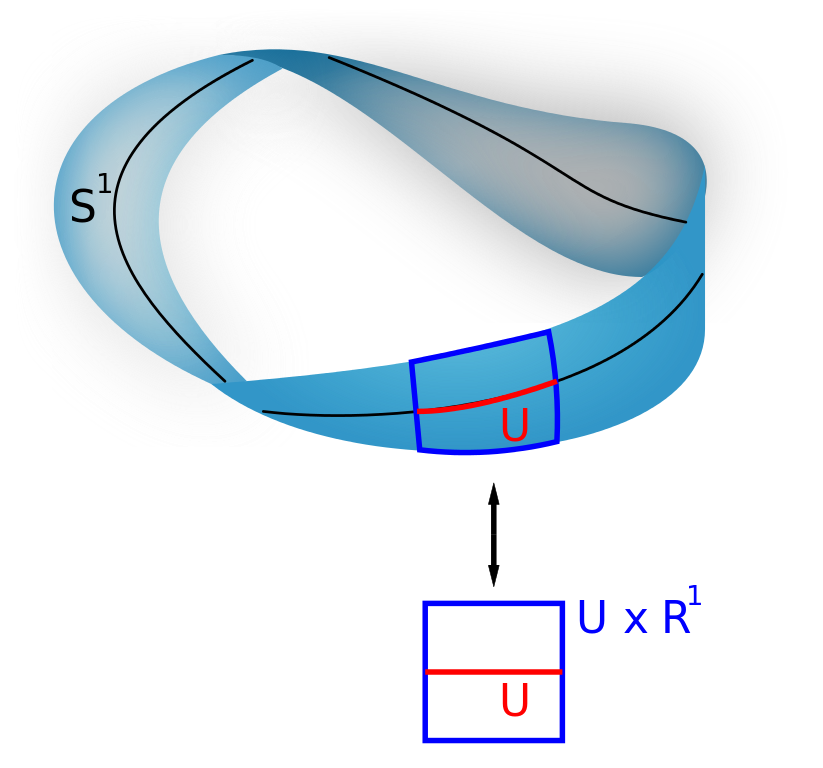In this example the vector bundle is the Möbius strip. The space $$X$$ is the circle $$S^1$$, and the vector space at each point is the line $$\mathbb{R}^1$$. If you restrict attention to a little open set $$U$$ near a point, the Möbius strip looks like $$U \times \mathbb{R}^1$$. So, you can say that localizing this vector bundle at any point gives $$\mathbb{R}^1$$.

But this nice easy-to-visualize stuff is also commutative algebra! For any compact Hausdorff space $$X$$, the continuous complex-valued functions on it, $$C(X)$$, form a kind of commutative ring called a 'commutative C*-algebra'. And any commutative C*-algebra comes from some compact Hausdorff space $$X$$. This fact is called the Gelfand-Naimark theorem.

(I liked this sort of commutative algebra because it involved a lot of things like I knew how to visualize, like topology and analysis. Also, C*-algebras describe the observables in classical and quantum systems, with commutative ones describing the classical ones.)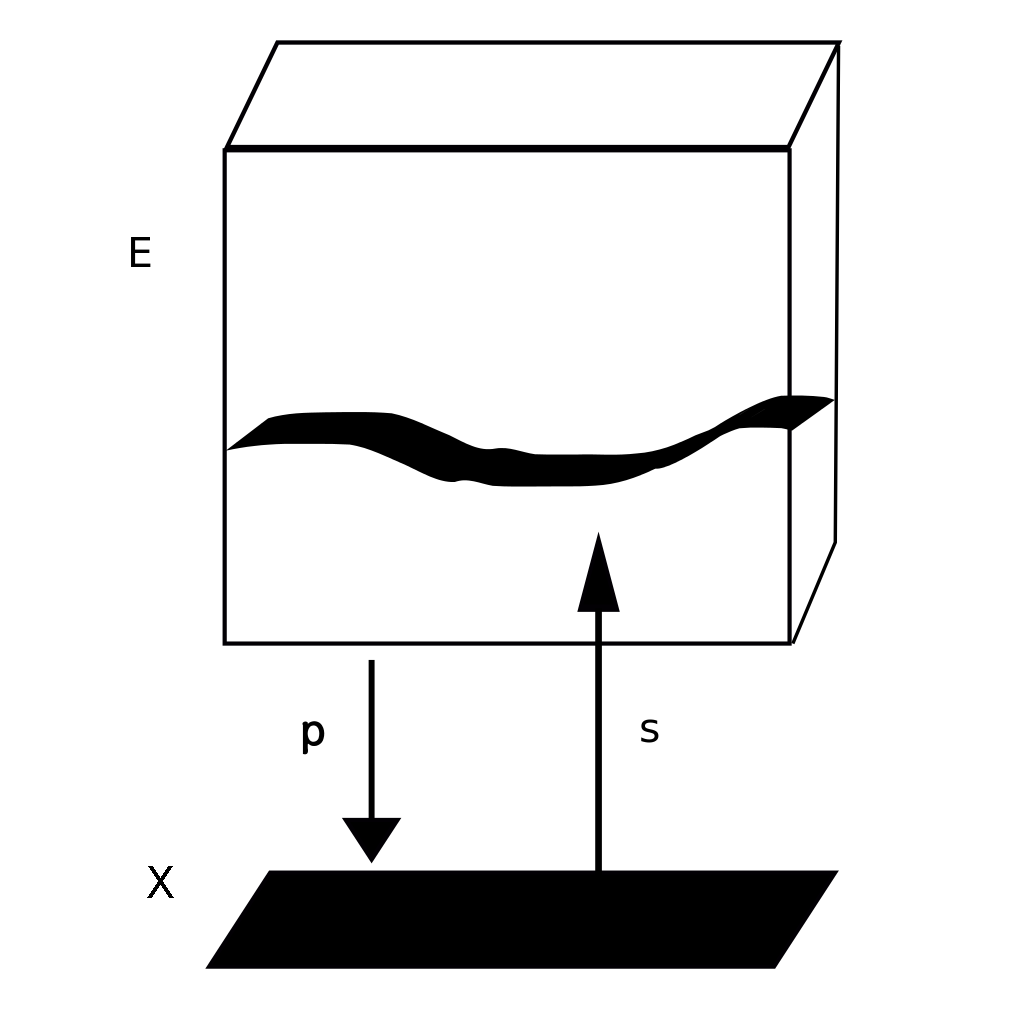Now, given a vector bundle over $$X$$, like $$E$$ here, its 'sections', like $$s$$ here, form a module of the commutative ring $$C(X)$$. And not just any sort of module: you get a 'finitely generated projective module'. (These are buzzwords that algebraists love.)

Again, we can turn this around: every finitely generated projective module of $$C(X)$$ comes from a vector bundle over $$X$$. This is called 'Swan's theorem'.

So: whenever someone says 'finitely generated projective module over a commutative ring' I think 'vector bundle' and see this:But to get this mental image to really do work for me, I had to learn how to 'localize' a projective module of a commutative ring 'at a point' and get something kind of like a vector space. And then I had to learn a bunch of basic theorems, so I could use this technology.

I could have learned this stuff in school like the other kids. I've sort of read about it anyway — you can't really avoid this stuff if you're in the math biz. But actually needing to do something with it radically increased my enthusiasm!

For example, I was suddenly delighted by Kaplansky's theorem. The analogue of a 'point' for a commutative ring $$R$$ is a prime ideal: an ideal $$\mathfrak{p} \subset R$$ that's not the whole ring such that $$ab \in \mathfrak{p}$$ implies either $$a \in \mathfrak{p}$$ or $$b \in \mathfrak{p}$$. We localize a ring $$R$$ at the prime ideal $$\mathfrak{p}$$ by throwing in formal inverses for all elements not in $$\mathfrak{p}$$. The result, called $$R_{\mathfrak{p}}$$, is a local ring, meaning a ring with just one maximal ideal.

When you do this, any module $$M$$ of $$R$$ gives a module $$M_{\mathfrak{p}}$$ of the localization of $$R$$. And Kaplansky's theorem says that any projective module of $$R$$ gives a free module of that local ring. This is a lot like a vector space over a field! After all, any vector space is a free module: this is just a fancy way of saying it has a basis.

Furthermore, a map between of modules of a commutative ring R has an inverse iff it's true 'localized at each point'. Just like a map between vector bundles over $$X$$ has an inverse iff the map between vector spaces it gives at each point of $$X$$ has an inverse!

I know all the algebraic geometers are laughing at me like a 60-year-old who just learned how to ride a tricycle and is gleefully rolling around the neighborhood. But too bad! It's never too late to have some fun!By the way, the quotes above come from this free book, which I'm enjoying now:

#### October 5, 2021

Stirling's formula gives a good approximation of the factorial $$n! = 1 \times 2 \times 3 \times \cdots \times n$$ It's obvious that $$n!$$ is smaller than $$n^n = n \times n \times n \times \cdots \times n$$ But where do the $$e$$ and $$\sqrt{2 \pi}$$ come from?

The easiest way to see where the $$\sqrt{2 \pi}$$ comes from is to find an integral that equals n! and then approximate it with a 'Gaussian integral', shown below.

This is famous: when you square it, you get an integral with circular symmetry, and the $$2 \pi$$ pops right out!

But how do you get an integral that equals $$n$$ factorial? Try integrating $$x^n$$ times an exponential! You have to integrate this by parts repeatedly. Each time you do, the power of $$x$$ goes down by one and you can pull out the exponent: first $$n$$, then $$n-1$$, then $$n-2$$, etc.

Next, write $$x^n$$ as $$e^{n \ln x|$$. With a little cleverness, this gives a formula for n! that's an integral of $$e$$ to $$n$$ times something. This is good for seeing what happens as $$n \to \infty$$.

There's just one problem: the 'something' also involves $$n$$: it contains $$\ln(ny)$$.

But we can solve this problem by writing $$\ln(ny) = \ln n + \ln y$$. With a little fiddling this gives an integral of $$e$$ to $$n$$ times something that doesn't depend on $$n$$. Then, as we take $$n \to \infty$$, this will approach a Gaussian integral. And that's why $$\sqrt{2 \pi}$$ shows up!

Oh yeah — but what about proving Stirling's formula? Don't worry, this will be easy if we can do the hard work of approximating that integral. It's just a bit of algebra:

So, this proof of Stirling's formula has a 'soft outer layer' and a 'hard inner core'. First you did a bunch of calculus tricks. But now you need to take the $$n \to \infty$$ limit of the integral of $$e$$ to $$n$$ times some function.

Luckily you have a pal named Laplace....

Laplace's method is not black magic. It amounts to approximating your integral with a Gaussian integral, which you can do by hand. Physicists use this trick all the time! And they always get a factor of $$\sqrt{2 \pi}$$ when they do this.

You can read more details here:

But in math, there are always mysteries within mysteries. Gaussians show up in probability theory when we add up lots of independent and identically distributed random variables. Could that be going on here somehow?

Yes! See this:

Folks at the n-Category Café noticed more mysteries. $$n!/n^n$$ is the probability that a randomly chosen function from an $$n$$-element set to itself is a permutation. Stirling's formula is a cool estimate of this probability! Can we use this to prove Stirling's formula? I don't know!

So I don't think we've gotten to the bottom of Stirling's formula! Comments at the n-Category Café contain other guesses about what it might 'really mean'. But they haven't crystallized yet.

#### October 7, 2021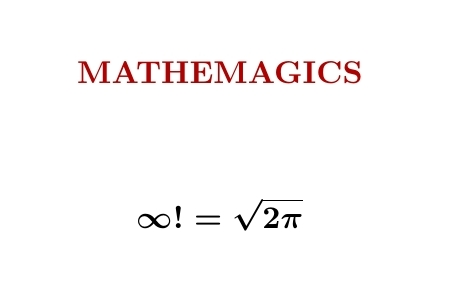'Mathemagics' is a bunch of tricks that go beyond rigorous mathematics. Particle physicists use them a lot. Using a mathemagical trick called 'zeta function regularization', we can 'show' that infinity factorial is $$\sqrt{2 \pi}$$.

This formula doesn't make literal sense, but we can write $$\ln (\infty!) = \sum_{n = 1}^\infty \ln n .$$ This sum diverges, but $$\sum_{n = 1}^\infty n^{-s} \ln n$$ converges for $$\mathrm{Re}(s) > 1$$ and we can analytically continue this function to $$s = 0$$, getting $$\frac{1}{2} \ln 2 \pi$$. So using this trick we can argue that $$\ln(\infty!) = \frac{1}{2} \ln 2 \pi,$$ giving the equation above. Don't take it too seriously... but physicists use tricks like this all the time, and get results that agree with experiment.

To understand this trick we need to notice that $$\sum_{n = 1}^\infty n^{-s} \ln n = - \frac{d}{ds} \zeta(s)$$ where $$\zeta(s) = \sum_{n = 1}^\infty n^{-s}$$ is the definition of the Riemann zeta function for $$\mathrm{Re}(s) > 1$$. But then — the hard part — we need to show we can analytically continue the Riemann zeta function to $$s = 0$$, and get $$\zeta'(0) = -\frac{1}{2} \ln 2 \pi$$ This last fact is a spinoff of Stirling's formula $$n! \sim \sqrt{2 \pi n} \,\left( \frac{n}{e} \right)^n$$ So the mathemagical formula for $$\infty!$$ is a crazy relative of this well-known, perfectly respectable asymptotic formula for $$n!$$.

He argues that today's mathemagics can become tomorrow's mathematics.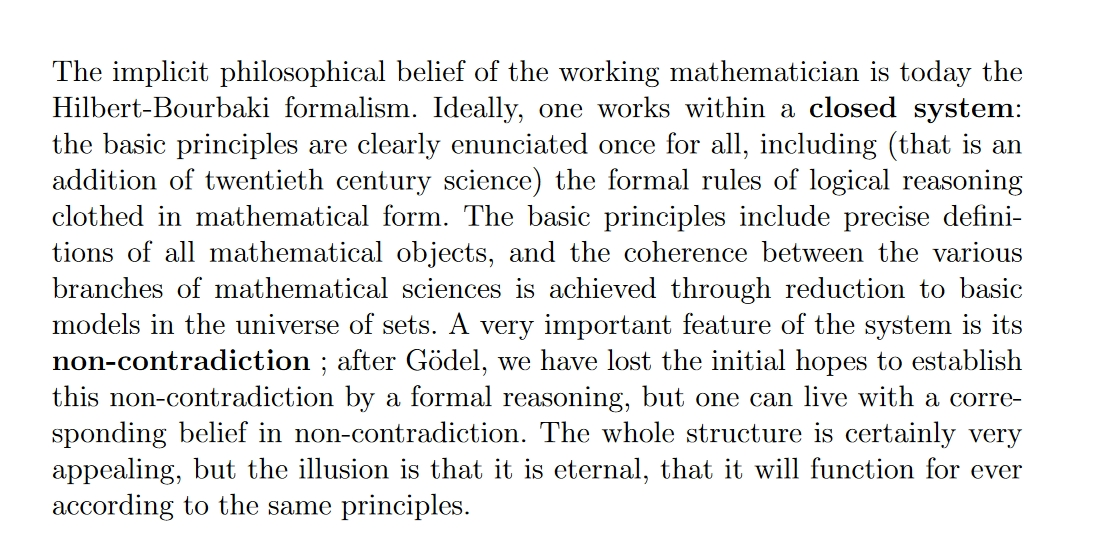#### October 8, 2021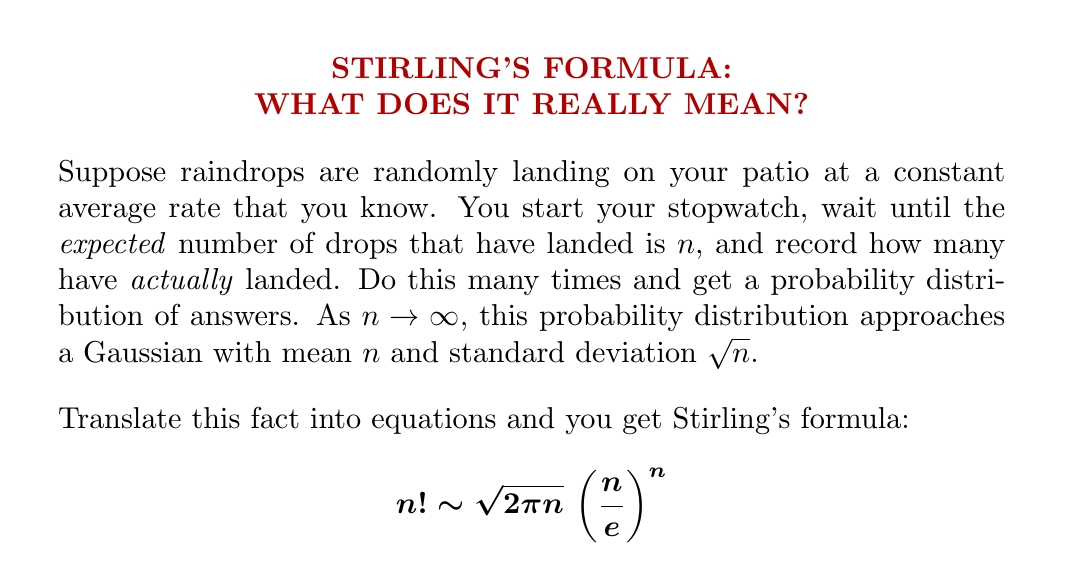Stirling's formula for the factorial looks cool — but what does it really mean? This is my favorite explanation. You don't see the numbers $$e$$ and $$2\pi$$ in the words here, but they're hiding in the formula for a Gaussian probability distribution!

My description in words is informal. I'm really talking about a Poisson distribution. If raindrops land at an average rate $$r$$, this says that after time $$t$$ the probability of $$k$$ having landed is $$\frac{(rt)^k e^{-rt}}{k!}$$ This is where the factorial comes from.

At time $$t$$, the expected number of drops to have fallen is clearly $$rt$$. Since I said "wait until the expected number of drops that have landed is $$n$$", we want $$rt = n$$. Then the probability of $$k$$ having landed is $$\frac{n^k e^{-n}}{k!}$$

Next, what's the formula for a Gaussian with mean $$n$$ and standard deviation $$\sqrt{n}$$? Written as a function of $$k$$, it's

$$\frac{e^{-(k-n)^2/2n}}{\sqrt{2 \pi n}}$$ If this matches the Poisson distribution above in the limit of large $$n$$, the two functions must match when $$k = n$$, at least asymptotically, so $$\frac{n^n e^{-n}}{n!} \sim \frac{1}{\sqrt{2 \pi n}}$$ And this becomes Stirling's formula after a tiny bit of algebra!

I learned about this on Twitter: Ilya Razenshtyn showed how to prove Stirling's formula starting from probability theory this way. But it's much easier to use his ideas to check that my paragraph in words is a way of saying Stirling's formula.

#### October 13, 2021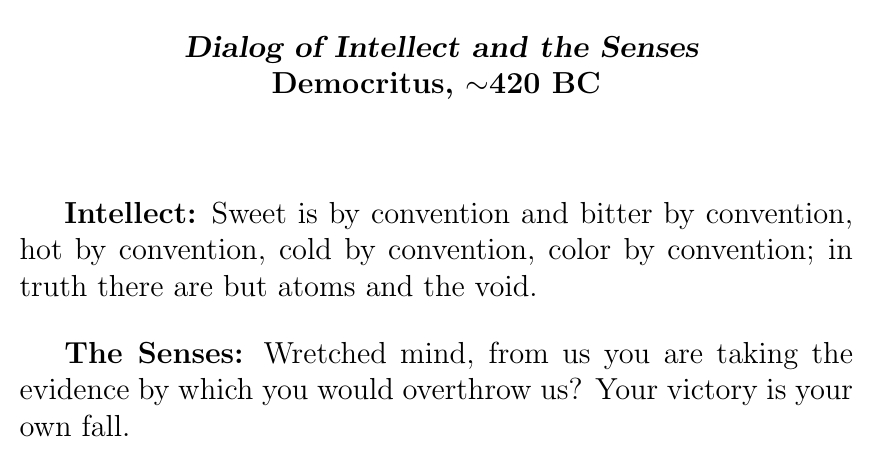They called Democritus 'the laughing philosopher'. He not only came up with atoms: he explained how weird it is that science, based on our senses, has trouble explaining what it feels like to sense something.

And he did it in a way that would make a great comedy routine with two hand puppets.

By the way: a lot of my diary entries these days are polished versions of my tweets. Yesterday Dominic Cummings retweeted the above tweet of mine. He was chief advisor to Boris Johnson for about a year, and on Twitter he loves to weigh in on the culture wars, on the conservative side.It's not as weird as when Ivanka Trump liked my tweet about rotations in 4 dimensions, but still it's weird. Or maybe not: Patrick Wintour in The Guardian reported that "Anna Karenina, maths and Bismarck are his three obsessions." But I wish some nice bigshots would like or retweet my stuff.

#### October 16, 2021

I love this movie showing a solution of the Kuramoto–Sivashinsky equation, made by Thien An. If you haven't seen her great math images on Twitter, check them out!

I hadn't known about this equation, and it looked completely crazy to me at first. But it turns out to be important, because it's one of the simplest partial differential equations that exhibits chaotic behavior.

As the image scrolls to the left, you're seeing how a real-valued function $$u(t,x)$$ of two real variables changes with the passage of time. The vertical direction is 'space', $$x$$, while the horizontal direction is time, $$t$$.

As time passes, bumps form and merge. I conjecture that they don't split or disappear. This reflects the fact that the Kuramoto–Sivashinsky equation has a built-in arrow of time: it describes a world where the future is different than the past.

The behavior of these bumps makes the Kuramoto–Sivashinsky equation an excellent playground for thinking about how differential equations can describe 'things' with some individuality, even though their solutions are just smooth functions. I'm going to make some conjectures about them. But I could really use some help from people who are good at numerical computation or creating mathematical images!

First let me review some known stuff.

For starters, note that where these bumps form is hard to predict: they seem to appear out of nowhere. That's because this system is chaotic: small ripples get amplified. This is especially true of ripples with a certain wavelength: roughly $$2 \sqrt{2} \pi$$, as we'll see later.

And yet while solutions of the Kuramoto–Sivanshinsky equation are chaotic, they have a certain repetitive character. That is, they don't do completely novel things; they seem to keep doing the same general sort of thing. The world this equation describes has an arrow of time, but it's ultimately rather boring compared to ours.

The reason is that all smooth solutions of the Kuramoto–Sivanshinsky equation quickly approach a certain finite-dimensional manifold of solutions, called an 'inertial manifold'. The dynamics on the inertial manifold is chaotic. And sitting inside it is a set called an 'attractor', which all solutions approach. This attractor is probably a fractal. This attractor describes the complete repertoire of what you'll see solutions do if you wait a long time.

Some mathematicians have put a lot of work into proving these things, but let's see how much we can understand without doing anything too hard.

Written out with a bit less jargon, the Kuramoto–Sivashinky equation says $$\displaystyle{ \frac{\partial u}{\partial t} = - \frac{\partial^2 u}{\partial x^2} - \frac{\partial^4 u}{\partial x^4} - \frac{1}{2}\left( \frac{\partial u}{\partial x}\right)^2 }$$

or in more compressed notation, $$u_t = -u_{xx} -u_{xxxx} - (u_x)^2$$ To understand it, first remember the heat equation: $$u_t = u_{xx}$$ This describes how heat spreads out. That is: if $$u(t,x)$$ is the temperature of an iron rod at position $$x$$ at time $$t$$, the heat equation describes how this temperature function flattens out as time passes and heat spreads.

But the Kuramoto–Sivashinsky equation more closely resembles the time-reversed heat equation $$u_t = -u_{xx}$$ This equation describes how, running a movie of a hot iron rod backward, heat tends to bunch up rather than smear out! Small regions of different temperature, either hotter or colder than their surroundings, will tend to amplify.

This accounts for the chaotic behavior of the Kuramoto–Sivashinsky equation: small bumps emerge as if out of thin air and then grow larger. But what keeps these bumps from growing uncontrollably?

The next term in the equation helps. If we have $$u_t = -u_{xx} - u_{xxxx}$$ then very sharp spikes in $$u(t,x)$$ tend to get damped out exponentially.

To see this, it helps to bring in a bit more muscle: Fourier series. We can easily solve the heat equation if our iron rod is the interval $$[0,2\pi]$$ and we demand that its temperature is the same at both ends: $$u(t,0) = u(t,2\pi)$$ This lets us write the temperature function $$u(t,x)$$ in terms of the functions $$e^{ikx}$$ like this: $$\displaystyle{ u(t,x) = \sum_{k = -\infty}^\infty \hat{u}_k(t) e^{ikx} }$$ for some functions $$\hat{u}_k(t)$$. Then the heat equation gives $$\displaystyle{ \frac{d}{d t} \hat{u}_k(t) = -k^2 \hat{u}_k(t) }$$ and we can solve these equations and get $$\displaystyle{ \hat{u}_k(t) = e^{-k^2 t} \hat{u}_k(0) }$$ and thus $$\displaystyle{ u(t,x) = \sum_{k = -\infty}^\infty \hat{u}_k(0) e^{-k^2 t} e^{ikx} }$$ So, each function $$\hat{u}_k(t)$$ decays exponentially as time goes on, and the so-called 'high-frequency modes', $$\hat{u}_k(t)$$ with $$|k|$$ big, get damped really fast due to that $$e^{-k^2 t}$$ factor. This is why heat smears out as time goes on.

If we solve the time-reversed heat equation the same way we get $$\displaystyle{ u(t,x) = \sum_{k = -\infty}^\infty \hat{u}_k(0) e^{k^2 t} e^{ikx} }$$ so now high-frequency modes get exponentially amplified. The time-reversed heat equation is a very unstable: if you change the initial data a little bit by adding a small amount of some high-frequency function, it will make an enormous difference as time goes by.

What keeps things from going completely out of control? The next term in the equation helps: $$u_t = -u_{xx} - u_{xxxx}$$ This is still linear so we can still solve it using Fourier series. Now we get $$\displaystyle{ u(t,x) = \sum_{k = -\infty}^\infty \hat{u}_k(0) e^{(k^2-k^4) t} e^{ikx} }$$ Since $$k^2 - k^4 \le 0$$, none of the modes $$\hat{u}_k(t)$$ grows exponentially. In fact, all the modes decay exponentially except for three: $$k = -1,0,1$$. These will be constant in time. So, any solution will approach a constant as time goes on!

We can make the story more interesting if we don't require our rod to have length $$2\pi$$. Say it has length $$L$$. We can write functions on the interval $$[0,L]$$ as linear combinations of functions $$e^{ikx}$$ where now the frequencies $$k$$ aren't integers: instead $$k = 2\pi n/L$$ for integers $$n$$. The longer our rod, the lower these frequencies $$k$$ can be. The rest of the math works almost the same: we get $$\displaystyle{ u(t,x) = \sum_{n = -\infty}^\infty \hat{u}_k(0) e^{(k^2-k^4) t} e^{ikx} }$$ but we have to remember $$k = 2\pi n/L$$. The modes with $$k^2 - k^4 > 0$$ will grow exponentially, while the rest will decay exponentially or stay constant. Note that $$k^2 - k^4 > 0$$ only for $$0 <|k| < 1$$. So, modes with these frequencies grow exponentially. Modes with $$|k| > 1$$ decay exponentially.

If $$L < 2\pi$$, all the frequencies $$k$$ are integers times $$2\pi/L$$, which is bigger than $$1$$, so no modes grow exponentially — and indeed all solutions approach a constant! But as you look at longer and longer rods, you get more and more modes that grow exponentially. The number of these will be roughly proportional to $$L$$, though they will 'pop into existence' at certain specific values of $$L$$.

Which exponentially growing modes grow the fastest? These are the ones that make $$k^2 - k^4$$ as large as possible, so they happen near where $$\displaystyle{ \frac{d}{dk} (k^2 - k^4) = 0 }$$ namely $$k = 1/\sqrt{2}$$. The wavelength of a mode is $$2\pi/k$$, so these fastest-growing modes have wavelength close to $$2\sqrt{2} \pi$$.

In short, our equation has a certain length scale where the instability is most pronounced: temperature waves with about this wavelength grow fastest.

All this is very easy to work out in as much detail as we want, because our equation so far is linear. The full-fledged Kuramoto–Sivashinsky equation $$u_t = -u_{xx} - u_{xxxx} - (u_x)^2$$ is a lot harder. And yet some features remain, which is why it was worth spending time on the linear version.

For example, it turns out that the bumps we see in the movie above have width roughly $$2 \sqrt{2} \pi$$. Bumps of roughly this width tend to get amplified. Why don't they keep on growing forever? Apparently the nonlinear term $$-(u_x)^2$$ prevents it. But this is not obvious. Indeed, it's conjectured that if you solve the Kuramoto–Sivashinsky equation starting with a bounded smooth function $$u(0,x)$$, the solution will remain bounded by a constant. But this has not been proved — or at least it was not proved as of 2000, when this very nice article was written:

The most fascinating fact about the Kuramoto–Sivashinsky equation is that for any fixed length $$L$$, it has a finite-dimensional manifold $$M$$ of solutions such that every solution approaches one of these, exponentially fast! So, while this equation really describes an infinite-dimensional dynamical system, as $$t \to \infty$$ its solutions move closer and closer to the solutions of some finite-dimensional dynamical system. This finite-dimensional system contains all the information about the patterns we're seeing in Thien An's movie.

As I mentioned, the manifold $$M$$ is called an 'inertial manifold'. This is a general concept in dynamical systems theory:

To make these ideas precise we need to choose a notion of distance between two solutions at a given time. A good choice uses the $$L^2$$ norm for periodic functions on $$[0,L]$$: $$\|f\| = \sqrt{\int_0^L |f(x)|^2 \, dx}.$$ Functions on $$[0,L]$$ with finite $$L^2$$ norm form a Hilbert space called $$L^2[0,L]$$. If we start with any function $$u(0,-)$$ in this Hilbert space we get a solution $$u(t,x)$$ of the Kuramoto–Sivashinsky equation such that the function $$u(t,-)$$ is in this Hilbert space at all later times $$t$$. Furthermore, this function is smooth, even analytic, for all later times:
This smoothing property is well-known for the heat equation, but it's much less obvious here!

This work also shows that the Kuramoto–Sivashinsky equation defines a dynamical system on the Hilbert space $$L^2[0,\infty]$$. And based on earlier work by other mathematicians, Temam and Wang have heroically shown that this Hilbert space contains an inertial manifold of dimension bounded by some constant times $$L^{1.64} (\ln L)^{0.2}.$$

I conjecture that in reality its dimension grows roughly linearly with $$L$$. Why? We've just seen this is true for the linearized version of the Kuramoto–Sivashinsky equation: all modes with frequency $$|k| > 1$$ get damped exponentially, but since there's one mode for each integer $$n$$, and $$k = 2\pi n/L$$, these modes correspond to integers $$n$$ with $$|n| \le L /2\pi$$. So, there are $$\lfloor L/\pi \rfloor$$ of these modes. In short, for the linearized Kuramoto–Sivashinsky equation the inertial manifold has dimension about $$L/\pi$$.

This evidence is rather weak, since it completely ignores the nonlinearity of the Kuramoto–Sivashinsky equation. I would not be shocked if the dimension of the inertial manifold grew at some other rate than linearly with $$L$$.

Sitting inside the inertial manifold is an attractor, the smallest set that all solutions approach. This is probably a fractal, since that's true of many chaotic systems. So besides trying to estimate the dimension of the inertial manifold, which is an integer we should try to estimate the dimension of this attractor, which may not be an integer!

There have been some nice numerical experiments studying solutions of the Kuramoto–Sivashinsky equation for various values of $$L$$, seeing how they get more complicated as $$L$$ increases. For small $$L$$, every solution approaches a constant, just as in the linearized version. For larger $$L$$ we get periodic solutions, and as $$L$$ continues to increase we get period doubling and finally chaos — a typical behavior for dynamical systems. But that's just the start. For details, read this:

I'll warn you that they use a slightly different formalism. Instead of changing the length $$L$$, they keep it equal to $$2\pi$$ and change the equation, like this: $$u_t = -u_{xx} - v u_{xxxx} - (u_x)^2$$ for some number $$v$$ they call the 'viscosity'. It's just a different way of describing the same business, so if I had more energy I could figure out the relation between $$L$$ and $$v$$ and tell you at which length $$L$$ chaos first kicks in. But I won't now.

Instead, I want to make some conjectures. I believe there is some fairly well-defined notion of a 'bump' for the Kuramoto–Sivashinsky equations: you can see the bumps form and merge here, and I believe there is a fairly simple way to actually count them at any moment in time:

Since we are studying solutions of the Kuramoto–Sivashinsky equation that are periodic in the space direction, we can think of space as a circle: the interval $$[0,L]$$ with its endpoints identified. Given a continuous solution $$u$$ of the equation, define a bump to be a maximal open interval in the circle such that $$u(t,x) > c$$ for all $$x$$ in this interval. This definition depends on a constant $$c > 0$$, but I think there is a range of values for which the following conjectures are true. One could guess this range with some numerical experiments, which I'm hoping one of you can do!

I believe that for nonnegative solutions in the inertial manifold, once a bump forms it never splits into two or more bumps or disappears: all it can do is merge with another bump.

I conjecture that if $$L$$ is large enough, almost every nonnegative solution on the inertial manifold has a finite number of bumps, and that while bumps can appear and merge, they can never split or disappear.

(Here 'almost every' is in the usual sense of measure theory. There are certainly solutions of the Kuramoto–Sivashinsky equation that don't have bumps that appear and merge, like constant solutions. These solutions may lie on the inertial manifold, but I'm claiming they are rare.)

I also conjecture that the time-averaged number of bumps is asymptotically proportional to $$L$$ as $$L \to \infty$$ for almost every nonnegative solution on the inertial manifold. The constant of proportionality shouldn't depend on the solution we pick, except for solutions in some set of measure zero. It will, however, depend on our constant $$c$$ that defines the minimum height of an official 'bump'.

I also conjecture that there's a well-defined time average of the rate at which new bumps form, which is also asymptotically proportional to $$L$$ and independent of which solution we pick, except for solutions in a set of measure zero.

I also conjecture that this rate equals the time-averaged rate at which bumps merge, while the time-averaged rate at which bumps disappear or split is zero.

These conjectures are rather bold, but of course there are various fallback positions if they fail.

How can we test these conjectures? It's hard to explicitly describe solutions that are actually on the inertial manifold, but by definition, any solution keeps getting closer to the inertial manifold at an exponential rate. Thus, it should behave similarly to solutions that are on the inertial manifold, after we wait long enough. So, I'll conjecture that the above properties hold not only for almost every solution on the inertial manifold, but for typical solutions that start near the inertial manifold... as long as we wait long enough when doing our time averages. This makes the conjectures testable with numerical experiments.

Here are some things I'd love to see:

• Movies like Thien An's, but with various choices of $$L.$$ To create these, maybe start with $$u(0,x) = \sin \frac{x}{2} + \textrm{a small amount of noise}$$ and run it for a longish time so it 'settles down' — that is, gets near the inertial manifold.
• More importantly: movies like the above, but where the solution is drawn in black if $$u(t,x) > c$$ and white otherwise. For a good choice of $$c$$ — and please tell me what choice that is! — we should see black stripes appear and merge but rarely if ever disappear or split.
• A time-averaged count of the average number of black stripes — that is, official 'bumps' — for various choices of $$L$$. I'm conjecturing that this is asymptotically proportional to $$L$$ for large $$L$$.
• Time-averaged counts of the rates at which black stripes are born, merge, split, and die — again for various choices of $$L.$$ I'm conjecturing that the first two are asymptotically proportional to $$L$$ for large $$L$$ and that they're equal. I'm conjecturing that the last two are zero, or tiny.
If someone gets into this, maybe we could submit a short paper to Experimental Mathematics. I've been browsing papers on the Kuramoto–Sivashinsky equations, and I haven't yet seen anything that gets into as much detail on what solutions look like as I'm trying to do here.

One last thing. I forgot to emphasize that the dynamical system on the Hilbert space $$L^2[0,L]$$ is not reversible: we can evolve a solution forwards in time and it will stay in this Hilbert space, but not backwards in time. This is very well-known for the heat equation; the point is that solutions get smoother as we run them forward, but when we run them backward they typically get more wild and eventually their $$L^2$$ norm blows up.

What makes this especially interesting is that the dynamical system on the inertial manifold probably is reversible. As long as this manifold is compact, it must be: any smooth vector field on a compact manifold $$M$$ generates a 'flow' that you can run forward or backward in time.

And yet, even if this flow is reversible, as I suspect it is, it doesn't resemble its time-reversed version! It has an 'arrow of time' built in, since bumps are born and merge much more often than they merge and split.

So, if my guesses are right, the inertial manifold for the Kuramoto–Sivashinsky equation describes a deterministic universe where time evolution is reversible — and yet the future doesn't look like the past, because the dynamics carry the imprint of the irreversible dynamics of the Kuramoto–Sivashinsky equation on the larger Hilbert space of all solutions.